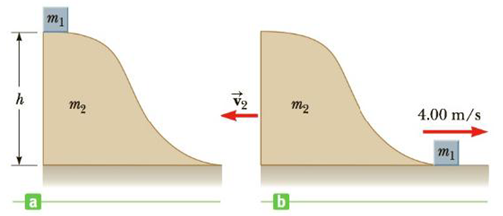Chapter 6, Problem 73AP

Chapter
Section
Textbook Problem

A small block of mass m1 = 0.500 kg is released from rest at the top of a curved wedge of mass m2 = 3.00 kg, which sits on a frictionless horizontal surface as in Figure P6.73a. When the block leaves the wedge, its velocity is measured to be 4.00 m/s to the right, as in Figure P6.73b. (a) What is the velocity of the wedge after the block reaches the horizontal surface? (b) What is the height h of the wedge?Figure P6.73

(a)

To determine
The velocity of the wedge after the block reaches the horizontal surface.

Explanation

The system has zero momentum initially and remains constant the motion. So, when m1 leaves, the equation is,

m2v2+m1v1=0v2=(m1m2)v1

• m1 is the mass of the block
• m2 is the mass of the wedge
• v1 is the velocity when the block leaves the wedge
• v2 is the velocity of the wedge

Substitute 0.500kg for m1 , 3

(b)

To determine
The height of the wedge.

Still sussing out bartleby?

Check out a sample textbook solution.

See a sample solution

The Solution to Your Study Problems

Bartleby provides explanations to thousands of textbook problems written by our experts, many with advanced degrees!

Get Started

Describe diffusion in terms or entropy.

Biology: The Unity and Diversity of Life (MindTap Course List)

The best and safest source of vitamin D for people in the United States is exposure to sunshine. T F

Nutrition: Concepts and Controversies - Standalone book (MindTap Course List)

Why are mathematical results rounded?

An Introduction to Physical Science

Name the following phenols.

Organic And Biological Chemistry